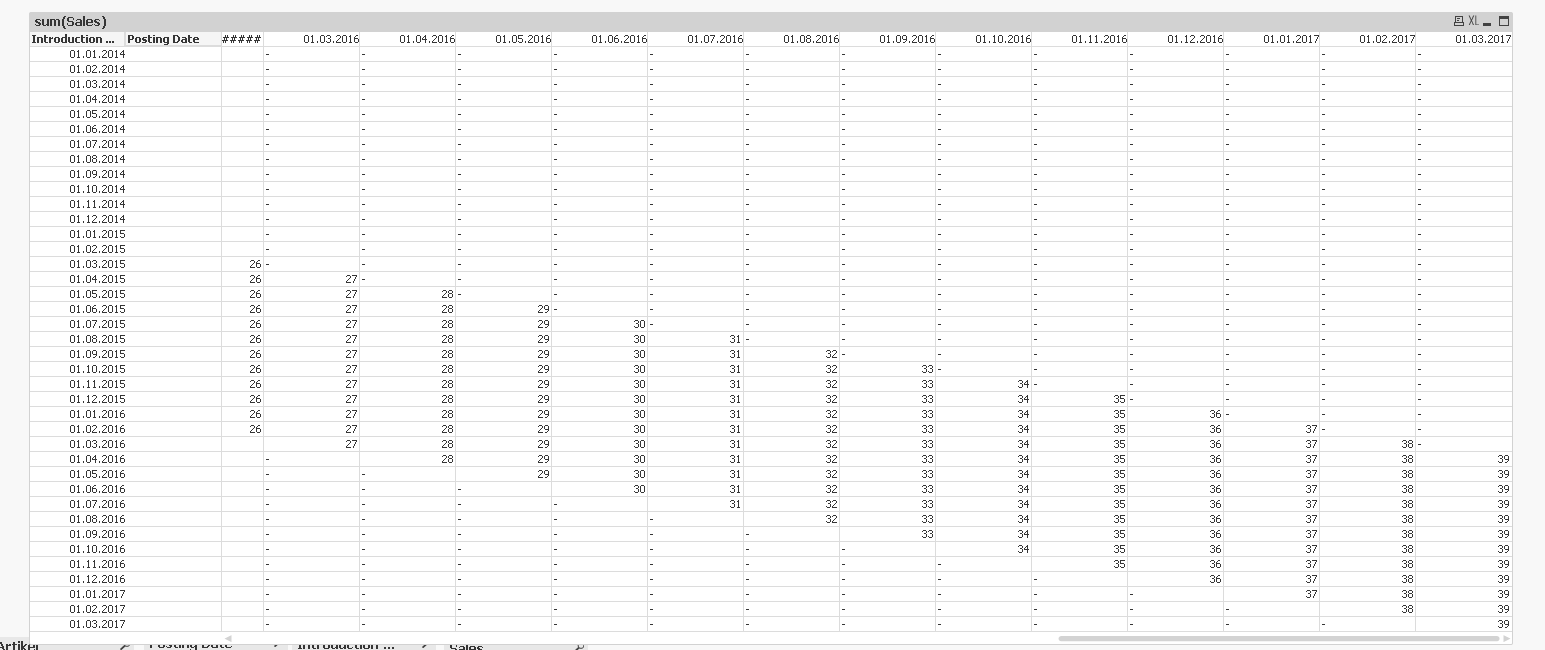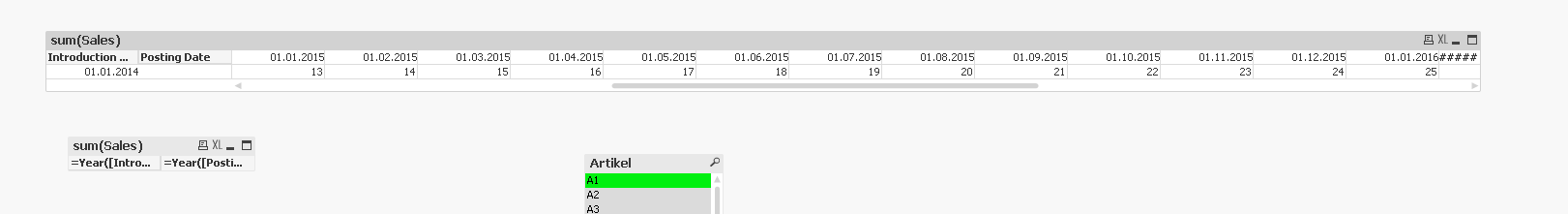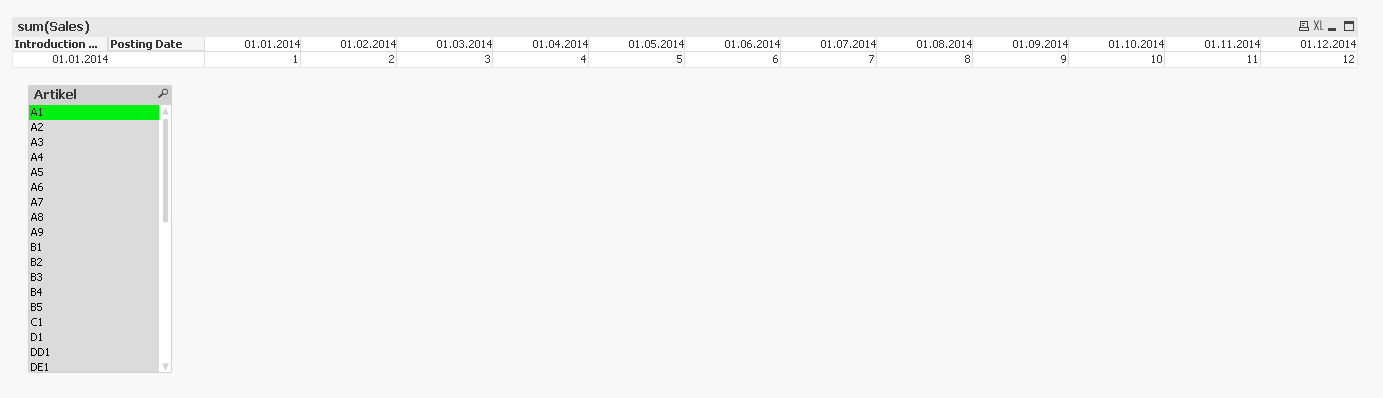# QlikView App Dev

Discussion Board for collaboration related to QlikView App Development.

Announcements
cancel
Showing results for
Did you mean:Contributor II

## Pivot Table Monthly Expression Date Range

Hi All,

I have pivot table monthly with filter Year = 2016 like in image attach.

I want to get sum sales value with date range each month.

Example :

Jan 2016, i want to get sum sales value from 1-Feb-2015 to 31-Jan-2016

Feb 2016, i want to get sum sales value from 1-Apr-2015 to 29-Feb-2016

I've tried this :

But it didn't get the right value.

Need your help for the expression

Many Thanks

12 RepliesMVP

May be this=If(Sum(TOTAL <[Posting Date]> Aggr(If(Sum(Sales) > 0, 1, 0), [Introduction Date], [Posting Date])) - RangeSum(Above(Sum(Aggr(If(Sum(Sales) > 0, 1, 0), [Introduction Date], [Posting Date])), 0, RowNo())) < 12, Sum(Sales))Contributor III

This is very strange.

In the  test application it is working, in my "big" application not...

I need to dig into it why.

Another question - if I select an article it seems not to work anymore:One article should be only visible during 12 months, but as soon as I select one article it is visible during the whole time when it has Sales...

Any idea how to restrict it to 12 months?MVP

Change the expression to this

=If(Sum(TOTAL <[Posting Date]> {<Artikel>} Aggr(If(Sum({<Artikel>}Sales) > 0, 1, 0), [Introduction Date], [Posting Date])) - RangeSum(Above(Sum({<Artikel>}Aggr(If(Sum({<Artikel>}Sales) > 0, 1, 0), [Introduction Date], [Posting Date])), 0, RowNo())) < 12, Sum(Sales))